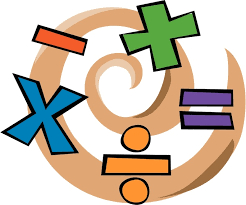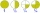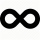# Quotient and product

If the quotient of [8/5 divided by 8/10] is added to the product of [8/14 x 7/12 x 3/8], what is the sum?

s =  0.125

### Step-by-step explanation:Did you find an error or inaccuracy? Feel free to write us. Thank you!Tips to related online calculators
Need help to calculate sum, simplify or multiply fractions? Try our fraction calculator.

## Related math problems and questions:

• Complicated sum minus productWhat must be subtracted from the sum of 3/8 and 5/16 to get difference equal to the product of 5/8 and 3/16?
• Quotient 3If the quotient of 8/13 and 2 is subtracted from the product of 1 3/4 and 8/21, what is the difference?
• Product increasedWhen the product of 2/3 and 6/10 is increased by 2/5, the result is?
• A quotientWhat is the quotient of 3/10 divided by 2/4 as a fraction?
• Quotient and divisionWhat is the quotient of 2/9 and 1/8?
• Numbers divisionWith what number should be divided mixed number 2 3/4 to get 11/12?
• Division by zeroFraction 5 by 2. if 3 is added to numerator and 2 is subtracted from the denominator then the new fraction is:
• The sum 12The sum of 3 mixed numbers is 20 13/15. two of the numbers are 6 1/3 and 7 5/6. what is the third number?
• Two numbers and its productThe product of two numbers are 2/3. If on of them is 1/10, what is the other?
• Divide 11Divide the product of 4 and 5/8 by 1 1/2. Write your answer as a mixed number.
• Evaluate expressionEvaluate expression using BODMAS rule: 1 1/4+1 1/5÷3/5-5/8
• Evaluate fractionsThe difference  of 5 1/2 and 2/3 is added to the product of 5/6 and 1/27 is added to the sum of 4/5 and 6/7(a) Convert the following mixed numbers to improper fractions. i. 3 5/8 ii. 7 7/6 (b) Convert the following improper fraction to mixed number. i. 13/4 ii. 78/5 (c) Simplify these fractions to their lowest terms. i. 36/42 ii. 27/45 2. evaluate following exFor one product, 5/8 of the metal sheet are consumed, to the second 5/6 of remains. What part of the sheet metal is consumed for both products together?What is the sum of 2/3+3/5?Multiply the quotient of 6 and 2 by 3, then add 1. Add 1 and 6, then divide by 2 and multiply by 3. Divide 6 by the product of 2 and 3, then add 1.